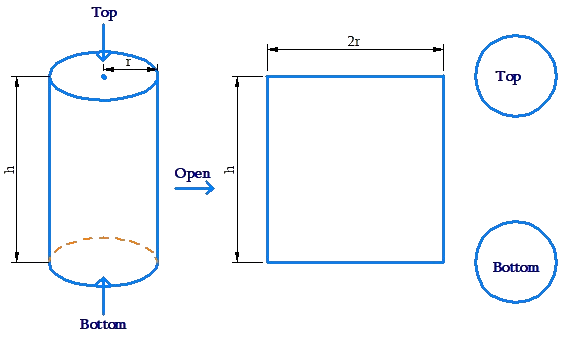# Surface Area of Cylinder

Surface Area of Cylinder
Go back to  'Surface Area'

The total surface area of the cylinder has two components:

• the curved surface area

• the flat surface area (of the two flat faces)

It is easy to see that the total flat surface area of the cylinder is $$2\pi {r^2}$$, because it is the sum of the areas of two circles. What about the curved surface area?

To determine that, suppose that we cut open the cylinder and roll it out into a planar rectangle:The height of this rectangle is the height of the cylinder h, while the length of this rectangle is the circumference of the face of the rectangle, that is, $$2\pi r$$. Thus, the area of this rectangle, and hence, the curved surface area of the cylinder, is $$2\pi rh$$.

To summarize:

• Flat SA= $$2\pi {r^2}$$

• Curved SA = $$2\pi rh$$

• Total SA = $$2\pi {r^2} + 2\pi rh = 2\pi r\left( {r + h} \right)$$

Mensuration and Solids
Mensuration and Solids
grade 10 | Questions Set 2
Mensuration and Solids